Scroll

# Improved Response (Category) Calculations

Follow
 Summary This article is one in a series of articles that will go through examples of using table arithmetic syntax new to Askia 5.3.5.0. This article shows the syntax available in the 'Calculation arithmetic' calculations and how to use it to work out an average. Applies to Askia Analyse. Written for Analysts, Researchers, Developers, Data Processors. Keywords Table arithmetic, Forloops, Coordinates, data, Calculation arithmetic, Average, Calculated response, Custom setting, Sub-population, Universe, Weighting, Level, Properties.

The .qes file and portfolio containing the example discussed is attached: Table Arithmetic Examples 5.rar

In Askia Analyse, you can add a calculated response to a categorical variable in rows or columns to perform calculations with the existing codes in this variable. You'll see an example below: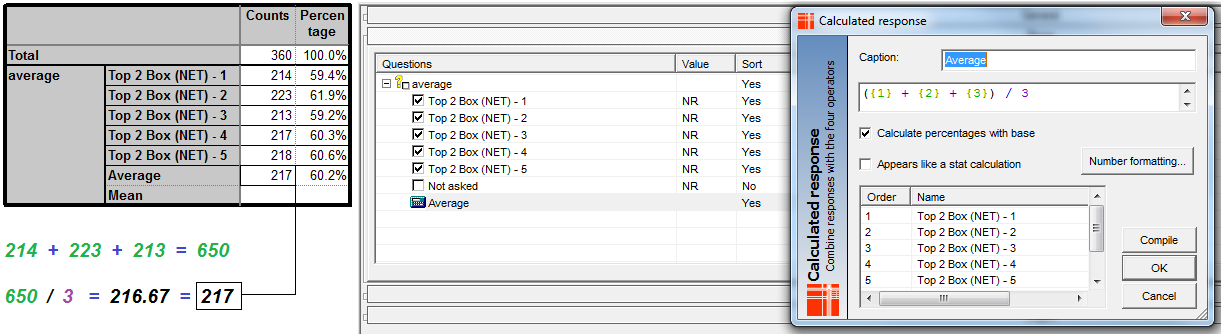However, if you change the custom settings of any of the first three codes in the example above, your average may no longer be correct. This is because calculated responses do not consider the custom settings applied to individual codes. i.e. if any of the below are changed: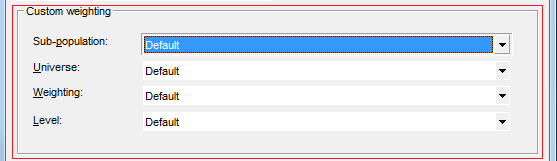If we do change the custom settings then we can work out our correct average by adding a calculation which uses table arithmetic. I have added the calculation ‘Mystuff’ in the example below where our variable now has a sub-population applied to each of the first three codes: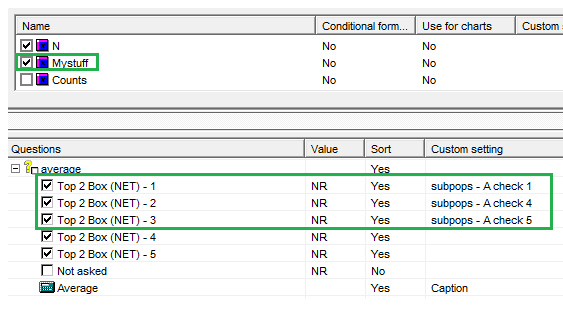In the screenshot below you can see the difference between how our old ‘Average’ calculated response behaves and how the new ‘Mystuff’ calculation behaves.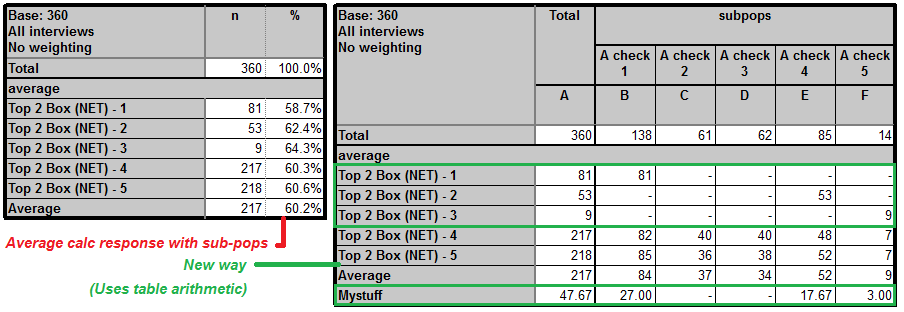The way we get this calculation to appear at the bottom of our table like a mean or median is be checking the box below 'Appears like a stat calculation'. This is seen below along with the syntax used to achieve our average.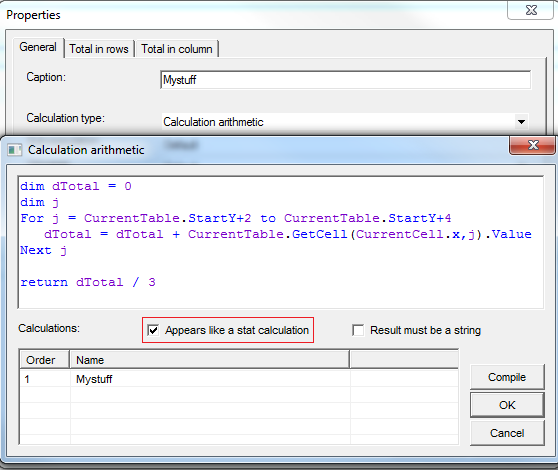There will be a more advanced example of this one to follow. It will go into detail on how to create an average of these three rows even if another category is inserted amongst them.